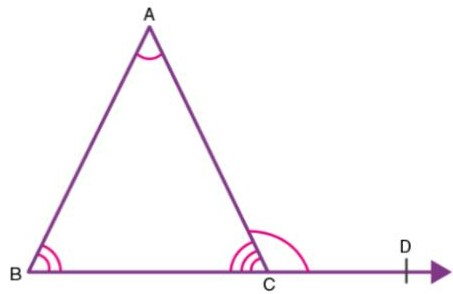Exterior angle property of a triangle

## Objective

To verify exterior angle property of a triangle.

## Exterior angle property of a triangle

The exterior angle theorem states that the measure of an exterior angle is equal to the sum of the measures of the two opposite (remote) interior angles of the triangle.

The exterior angle theorem is one of the most fundamental theorem of triangles. Before we begin with the details of the theorem, let see the basics of a Triangle.

### Triangle

A polygon is defined as a plane figure bounded by a finite number of line segments to form a closed figure. Triangle is the polygon bounded by a least number of line segments, i.e. three. It has three edges and three vertices.

Figure  below represents a triangle with three sides AB, BC, CA, and three vertices A, B and C.

∠ABC, ∠BCA and ∠CAB are the three interior angles of ∆ABC.If a side of a triangle is extented, then the exterior angle is formed.

In the figure above side BC is extended till D and the angle ∠ACD is the exterior angle.

According to the exterior angle property of a triangle theorem, the sum of measures of ∠ABC and ∠CAB would be equal to the exterior angle ∠ACD.

∠ABC + ∠CAB   =   ∠ACD